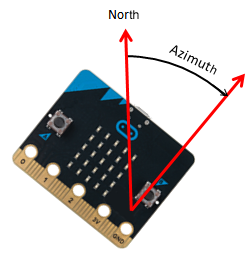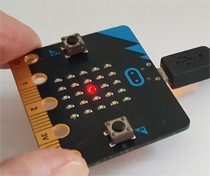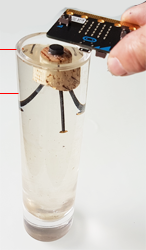kompass
 Deutsch   English   Français

## 6. MAGNETIC FIELD SENSOR, COMPASS### YOU LEARN HERE...

 how to measure the magnetic field with the micro:bit and determine the azimuth.

### CALIBRATE THE SENSOR AND DISPLAY VALUESYou can use the magnetic field sensor to determine the position of the micro:bit with respect to the north direction. To do this, use the command compass.heading(), which returns the azimuth in degrees (positive to the east) when the board is in a horizontal position.

 The sensor is clearly visible on your micro:bit (labeled COMPASS).However before you can start using the sensor, you must calibrate it. To do so, call compass.calibrate(). Then the program blocks and asks you to create a circle figure by tilting the board in all directions until all the outer LEDs form a glowing circle. To announce the end of the calibration process, the image HAPPY is shown and the program continues to run. Now you write out the azimuth as an integer between 0 and 360 in an endless loop.Program: ```from microbit import * compass.calibrate() while True: print(compass.heading()) sleep(300) ``` from microbit import * #compass.calibrate() while True: print(compass.heading()) sleep(300) ► Copy to clipboard

### EXAMPLES

Create a compass
 Like a real compass, an arrow should appear on the display pointing north. Since you can only show arrows in 45-degree directions, you divide the azimuth into 8 areas and assign the arrow that fits best to each area. If the sensor is not yet calibrated, you need to calibrate it first.Actually, you should also test for equality in the area boundaries. Since the sensor returns only integer values, you can do without it.

Program:

```from microbit import *

if not compass.is_calibrated():
compass.calibrate()

while True:
print(h)
if h > 22.5 and h < 67.5:
display.show(Image.ARROW_NW)
elif h > 67.5 and h < 112.5:
display.show(Image.ARROW_W)
elif h > 112.5 and h < 157.5:
display.show(Image.ARROW_SW)
elif h > 157.5 and h < 202.5:
display.show(Image.ARROW_S)
elif h > 202.5 and h < 247.5:
display.show(Image.ARROW_SE)
elif h > 247.5 and h < 292.5:
display.show(Image.ARROW_E)
elif h > 292.5 and h < 337.5:
display.show(Image.ARROW_NE)
else:
display.show(Image.ARROW_N)
sleep(10)
```
► Copy to clipboard

Search for mines
 Place a small magnet, as you find it for memo boards, under a cardboard cover. Imagine the magnet like a mine which somebody has to find using the micro:bit as a mine finder. In your program, the middle LED lights up the brighter the closer the board gets to the mine. You use  compass.get_values(), which returns the strength of the magnetic field as a tuple of the x, y, and z components.Then you calculate the magnitude from these three components and scale it to get a brightness value between 0 and 9.

Program:

```from microbit import *
import math

while True:
v = compass.get_values()
b = math.sqrt(v * v + v * v + v * v)
z = min(9, int(b / 500000)) # brightness
display.set_pixel(2, 2, z)
sleep(10)
```
► Copy to clipboard

Measure water level

Your test equipment consists of a flower vase and a magnet, which is attached to a floating cork. With three nails you stabilize the cork so that the magnet only moves in the vertical direction when the water level changes.

From the measurement of the magnitude of the magnetic field you get information about the height of the water column. You consider 3 water levels.It is best to imagine that the vessel is in three "states" that can take the values "high", "ok" and "low". From the scaled value of the magnetic field you make the decision whether the state changes. If you do not have sound, you may use a visible alarm on the LED display.Program: ```from microbit import * import math import music state = "ok" while True: v = compass.get_values() b = math.sqrt(v * v + v * v + v * v) z = int(b / 100000) if z >= 15 and state != "high": state = "high" print("->high") elif z >= 8 and z < 15 and state != "ok": state = "ok" print("->ok") elif z < 8 and state != "low": state = "low" print("->low") if state == "high": music.pitch(2000, 100) elif state == "low": music.pitch(500, 100) sleep(10) ``` from microbit import * import math import music state = "ok" while True: v = compass.get_values() b = math.sqrt(v * v + v * v + v * v) z = int(b / 100000) if z >= 15 and state != "high": state = "high" print("->high") elif z >= 8 and z < 15 and state != "ok": state = "ok" print("->ok") elif z < 8 and state != "low": state = "low" print("->low") if state == "high": music.pitch(2000, 100) elif state == "low": music.pitch(500, 100) sleep(10) ► Copy to clipboard

### MEMO

 The magnetic field sensor can detect the magnetic fields of a magnet or act as a compass instrument to find the direction with respect to the north direction. In this application, it must be calibrated first.

### EXERCISES

1.
 Build a "pirouette counter": Each time you turn around your own axis with the micro:bit in your hand, another LED on the display turns on. Instructions: When the micro:bit is spinning, compass.heading() will produce an irregular waveform.To count the rounds, a single switching level is not enough, but two levels must be defined. When the upper level is exceeded, the counter is increased and at the same time the counting is made inactive. Only when the measurement value goes below the lower level, the counting is activated again.
 2 Non-contact revolution counters (e.g. for rotating wheels) often use a magnet that passes in front of a magnetic field sensor once every revolution. Attach a magnet (for example from a memo board) to a bike wheel and write a program that writes out the number of revolutions in the terminal window. How can you use the concept to build an odometer?

6-1
##### Didactic hints:

The program can be written more elegantly by using a list of all 8 arrows and computing the angle ranges mathematically using the modulo operator. For students, this solution is more difficult to understand.

```from microbit import *

if not compass.is_calibrated():
compass.calibrate()

while True:
print(h)
h = (h - 22.5) % 360
i = 7 - int(h / 45)
display.show(Image.ALL_ARROWS[i])
sleep(100)
```
6-2
##### Didactic hints:

The solution shown here uses the programming paradigm "state programming", as it is widely used in conjuction with state machines.

It is important that only state changes are recorded. Programming with a multiple if-else structure is not self-evident and must be specially trained.

5-3
##### Professional hints:

In the well-tempered tuning the octave (doubling of the frequency) is divided into 12 semitones with the same frequency ratio r. So the following applies: r12 = 2 or
 r =5-1
##### Professional hints:

Some sound frequencies:

 Tone Frequency Tone Frequency
 h' 494 h'' 988 a' 440 a'' 880 g' 392 g'' 784 f' 349 f'' 698 e' 330 e'' 660 d' 294 d'' 588 c' 262 c'' 524 c''' 1048